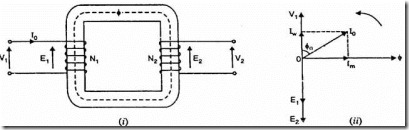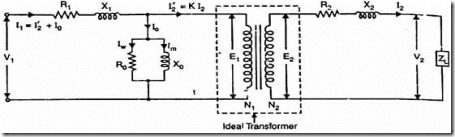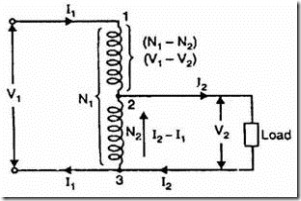### Transformers

Part A

1. What is step down transformer?

The transformer used to step down the voltage from primary to secondary is called as step down transformer. (Ex: 220/110V).

2. Draw the noload phasor diagram of a single phase transformer.3. Why is an auto-transformer not used as a distribution transformer?

The autotransformer cannot provide isolation between HV and LV side. Due to open circuit in the common portion, the voltage on the load side may soot up to dangerously high voltage causing damage to equipment. This unexpected rise in the voltage on LV side is potentially dangerous. Hence the autotransformer cannot be used as distribution transformer.

4. Give the basic principle behind the working of transformer.

The transformers works in the principle of mutual induction between two coils which are electrically isolated but magnetically coupled.

5. What are the conditions for parallel operation of transformer?

In order that the transformers work satisfactorily in parallel, the following conditions should be satisfied:

ñ Transformers should be properly connected with regard to their polarities.

ñ The voltage ratings and voltage ratios of the transformers should be the same.

ñ The per unit or percentage impedances of the transformers should be equal.

ñ The reactance/resistance ratios of the transformers should be the same.

6. What are the no load losses in a two winding transformer? And state the reasons for such losses.

The noload losses in transformer are,

ñ Hysteresis Loss: To establish the magnetic circuit in the transformer core.

ñ Eddy current loss: Due to circulation of current induced in the core due to induction.

7. Why is transformer rated in kVA?

The copper loss of a transformer depends on current and iron loss on voltage. Hence, total transformer loss depends on volt-ampere (VA) and not on phase angle between

voltage and current i.e., it is independent of load power factor. That’s why rating of transformers are in kVA and not in kW.

8. Compare two winding transformer and auto-transformer.

 Particulars Two Winding Transformer Auto Transformer No. Of windings Two windings One winding Output voltage Fixed unless tap changer is provided Variable voltage can be obtained Weight of Copper required More for two windings Less because of single winding Size Larger for same rating Small in size for same rating Efficiency Comparatively lesser Comparatively better

9. Classify the transformer according to the construction.

Depending upon the manner in which the primary and secondary are wound on the core, transformers are of two types viz., (i) core-type transformer and (ii) shell-type transformer.

10. What is transformation ratio?

It is the ratio in which the voltage to be transformed (stepped up or down) from primary to secondary of a transformer.

11. Draw the exact equivalent circuit of a transformer.12. What are the advantages of auto-transformer over ordinary transformer?

ñ The autotransformer is lesser size than ordinary two winding transformer for the same rating. Hence the cost reduced.

ñ Autotransformer operates at higher efficiency.

ñ Superior voltage regulation.

13. Mention the properties of oil used in transformers.

The following are the desirable properties of transformer oil:

· It should be free from moisture

· It should have high dielectric strength

· It should have thermally stability and higher thermal conductivity

· It should be contaminated by temperature rise.

14. Define voltage regulation of transformer.

The voltage regulation of a transformer is the arithmetic difference (not phasor difference) between the no-load secondary voltage (0V2) and the secondary voltage V2 on load expressed as percentage of no-load voltage.

15. Write down the volt-ampere transferred inductively and volt-ampere transferred conductively in an auto-transformer.Volt-ampere transferred inductively : V2(I2 – I1) Volt-ampere transferred conductively : KV1I1

16. What are the properties of an ideal transformer?

An ideal transformer has the following properties:

ñ No winding resistance

ñ No flux leakage

ñ No coreloss

ñ Magnetize at zero current

17. Mention the applications of auto-transformer.

The autotransformers are used in the following applications:

ñ To give small boost to a distribution cable to correct the voltage drop.

ñ As auto transformer starter to give upto 50% to 60% of full voltage to an induction motor during starting.

ñ As furnace transformers for getting a convenient supply to suit the furnace winding from a 230V supply.

ñ As interconnection transformers in 132kV/330kV system.

ñ In control equipment for single phase and three phase electrical locomotives.

18. Why V1:V2≠N1:N2 in a real (practical) transformer?

In practical transformers, the terminal output depends on the resistive drop and

magnetic leakages. Hence the ratio of turns do not match equal with the ratio of terminal voltages.

19. Explain the term percentage impedance as applied to transformer.

The percentage impedance is the per-unit impedance expressed as a percentage on a certain MVA and voltage base.

20. What are the various types of three phase transformer connections?

The most common types of transformer connections are,

i. Star-Star (Y-Y)

ii. Delta-Delta(∆-∆)

iii. Star-Delta (Y-∆)

21. What is an ideal transformer?

iv. Delta-Star (∆-Y)

v. Open Delta (V-V)

vi. Scott Connection (T-T)

The transformer has the following properties is said to be an ideal transformer:

ñ No winding resistance

ñ No flux leakage

ñ No coreloss

ñ Magnetize at zero current

In practical, it is difficult to satisfy all the above properties and the concept of ideal transformer is only an imaginative.

22. What are the two components of noload current in transformer?

The noload current contains two components as follows:

1. Loss component (Iw)

2. Magnetizing component (Im)

23. What is All day efficiency?

The ratio of output in kWh to the input in kWh of a transformer over a 24-hour period is known as all-day efficiency i.e.,

All day Efficiency = Output kWh for 24Hrs / Input kWh for 24Hrs

24. Define regulation and efficiency of a transformer.

The voltage regulation of a transformer is the arithmetic difference (not phasor difference) between the no-load secondary voltage (0V2) and the secondary voltage V2 on load expressed as percentage of no-load voltage

The efficiency of a transformer is defined as the ratio of output power (in watts or kW) to input power (watts or kW) i.e.,

h = Output Power  /Input Power

25. Mention the different losses in transformer.

The losses that occur in a transformer are:

(a) core losses—eddy current and hysteresis losses

Electrical Machines I : Transformers

(b) copper losses—in the resistance of the windings

26. Name the factors on which hysteresis loss depends.

1. Frequency, 2. Volume of the core, 3.Maximum flux density

PART – B

1. Explain how the efficiency of a transformer may be found from the open circuit and short circuit tests (16) (APR/MAY 2008)

(or)

Explain in detail the tests required to obtain the equivalent circuit parameters of transformer (8) (APR/MAY 2010).

(or)

Describe the method of calculating the regulation and efficiency of single phase transformer by OC and SC tests. (16) (May/June 2012)

2. Describe the constructional features of any one type of single phase transformer. (8) (APR/MAY 2008)

3. Show that the maximum efficiency in a transformer occurs when its variable loss is equal to constant loss. (6) (MAY/JUN 2007)

4. Explain in detail Eddy current loss. (5) (APR/MAY 2010)

5. Draw the equivalent circuit of single phase transformer and draw the necessary phasor diagram under resistive, inductive and capacitive loads. (8) (APR/MAY 2010)

(or)

Explain in detail step by step procedure to draw the equivalent circuit of transformer. (8) ((Nov/Dec 2012)

6. Explain in detail the various types of three phase transformer. (10) (APR/MAY 2010)

7. Prove that the amount of copper saved in autotransformer is (1-K) times of ordinary transformer. (6) (APR/MAY 2010)

(or)

Derive an expression for saving of copper when an autotransformer is used. (6) (Nov/Dec 2011)

8. Define Voltage regulation of a two winding transformer and explain its significance. (4) (Nov/Dec 2010)

9. Explain the reasons for tap changing in transformers. State on which winding the taps are provided and why? (4) (Nov/Dec 2010)

10. Explain clearly the causes of voltage drop in a power transformer on load and develop the equivalent circuit for a single phase transformer. (10) (Nov/Dec 2010)

11. Derive and expression for the emf of an ideal transformer. (6) (May/June 2012) (or)

Derive the emf equation of a transformer.(6) (Nov/Dec 2012)

12. Explain in detail about the parallel operation of transformers with equal and unequal voltage ratios . (16)